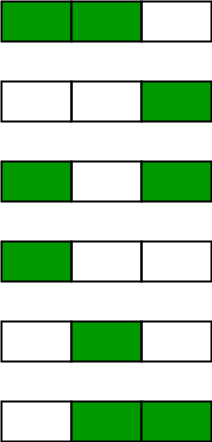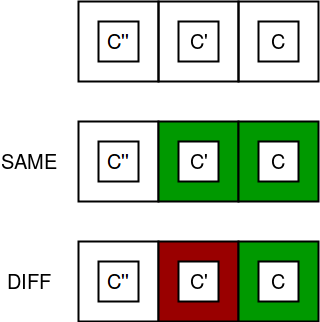# Painting Fence Algorithm

Given a fence with n posts and k colors, find out the number of ways of painting the fence such that at most 2 adjacent posts have the same color. Since answer can be large return it modulo 10^9 + 7.

Examples:

```Input : n = 2 k = 4
Output : 16
We have 4 colors and 2 posts.
Ways when both posts have same color : 4
Ways when both posts have diff color :
4*(choices for 1st post) * 3(choices for
2nd post) = 12

Input : n = 3 k = 2
Output : 6
```

## Recommended: Please solve it on “PRACTICE” first, before moving on to the solution.

Following image depicts the 6 possible ways of painting 3 posts with 2 colors:Consider the following image in which c, c’ and c” are respective colors of posts i, i-1 and i -2.According to the constraint of the problem, c = c’ = c” is not possible simultaneously, so either c’ != c or c” != c or both. There are k – 1 possibilities for c’ != c and k – 1 for c” != c.

``` diff = no of ways when color of last
two posts is different
same = no of ways when color of last
two posts is same
total ways = diff + sum

for n = 1
diff = k, same = 0
total = k

for n = 2
diff = k * (k-1) //k choices for
first post, k-1 for next
same = k //k choices for common
color of two posts
total = k +  k * (k-1)

for n = 3
diff = [k +  k * (k-1)] * (k-1)
(k-1) choices for 3rd post
to not have color of 2nd
post.
same = k * (k-1)
c'' != c, (k-1) choices for it

Hence we deduce that,
total[i] = same[i] + diff[i]
same[i]  = diff[i-1]
diff[i]  = (diff[i-1] + diff[i-2]) * (k-1)
= total[i-1] * (k-1)
```

Below is the implementation of the problem:

## C++

 `// C++ program for Painting Fence Algorithm ` `#include ` `using` `namespace` `std; ` ` `  `// Returns count of ways to color k posts ` `// using k colors ` `long` `countWays(``int` `n, ``int` `k) ` `{ ` `    ``// To store results for subproblems ` `    ``long` `dp[n + 1]; ` `    ``memset``(dp, 0, ``sizeof``(dp)); ` `    ``int` `mod = 1000000007; ` ` `  `    ``// There are k ways to color first post ` `    ``dp = k; ` ` `  `    ``// There are 0 ways for single post to ` `    ``// violate (same color_ and k ways to ` `    ``// not violate (different color) ` `    ``int` `same = 0, diff = k; ` ` `  `    ``// Fill for 2 posts onwards ` `    ``for` `(``int` `i = 2; i <= n; i++) ` `    ``{ ` `        ``// Current same is same as previous diff ` `        ``same = diff; ` ` `  `        ``// We always have k-1 choices for next post ` `        ``diff = dp[i-1] * (k-1); ` `        ``diff = diff % mod; ` ` `  `        ``// Total choices till i. ` `        ``dp[i] = (same + diff) % mod; ` `    ``} ` ` `  `    ``return` `dp[n]; ` `} ` ` `  `// Driver code ` `int` `main() ` `{ ` `    ``int` `n = 3, k = 2; ` `    ``cout << countWays(n, k) << endl; ` `    ``return` `0; ` `} `

## Java

 `// Java program for Painting Fence Algorithm ` `import` `java.util.*; ` ` `  `class` `GfG  ` `{ ` ` `  `// Returns count of ways to color k posts ` `// using k colors ` `static` `long` `countWays(``int` `n, ``int` `k) ` `{ ` `    ``// To store results for subproblems ` `    ``long` `dp[] = ``new` `long``[n + ``1``]; ` `    ``Arrays.fill(dp, ``0``); ` `    ``int` `mod = ``1000000007``; ` ` `  `    ``// There are k ways to color first post ` `    ``dp[``1``] = k; ` ` `  `    ``// There are 0 ways for single post to ` `    ``// violate (same color_ and k ways to ` `    ``// not violate (different color) ` `    ``int` `same = ``0``, diff = k; ` ` `  `    ``// Fill for 2 posts onwards ` `    ``for` `(``int` `i = ``2``; i <= n; i++) ` `    ``{ ` `        ``// Current same is same as previous diff ` `        ``same = diff; ` ` `  `        ``// We always have k-1 choices for next post ` `        ``diff = (``int``) (dp[i-``1``] * (k-``1``)); ` `        ``diff = diff % mod; ` ` `  `        ``// Total choices till i. ` `        ``dp[i] = (same + diff) % mod; ` `    ``} ` ` `  `    ``return` `dp[n]; ` `} ` ` `  `    ``// Driver code ` `    ``public` `static` `void` `main(String[] args) ` `    ``{ ` `        ``int` `n = ``3``, k = ``2``; ` `        ``System.out.println(countWays(n, k)); ` `    ``} ` `} ` ` `  `// This code contributed by Rajput-Ji `

Output:

```6
```

Space optimization :
We can optimize above solution to use one variable instead of a table.

Below is the the implementation of the problem:

## C++

 `// C++ program for Painting Fence Algorithm ` `#include ` `using` `namespace` `std; ` ` `  `// Returns count of ways to color k posts ` `// using k colors ` `long` `countWays(``int` `n, ``int` `k) ` `{ ` `    ``// There are k ways to color first post ` `    ``long` `total = k; ` `    ``int` `mod = 1000000007; ` ` `  `    ``// There are 0 ways for single post to ` `    ``// violate (same color_ and k ways to ` `    ``// not violate (different color) ` `    ``int` `same = 0, diff = k; ` ` `  `    ``// Fill for 2 posts onwards ` `    ``for` `(``int` `i = 2; i <= n; i++) ` `    ``{ ` `        ``// Current same is same as previous diff ` `        ``same = diff; ` ` `  `        ``// We always have k-1 choices for next post ` `        ``diff = total * (k-1); ` `        ``diff = diff % mod; ` ` `  `        ``// Total choices till i. ` `        ``total = (same + diff) % mod; ` `    ``} ` ` `  `    ``return` `total; ` `} ` ` `  `// Driver code ` `int` `main() ` `{ ` `    ``int` `n = 3, k = 2; ` `    ``cout << countWays(n, k) << endl; ` `    ``return` `0; ` `} `

## Java

 `// Java program for Painting Fence Algorithm ` `class` `GFG  ` `{ ` `     `  `// Returns count of ways to color k posts ` `// using k colors ` `static` `long` `countWays(``int` `n, ``int` `k) ` `{ ` `    ``// There are k ways to color first post ` `    ``long` `total = k; ` `    ``int` `mod = ``1000000007``; ` ` `  `    ``// There are 0 ways for single post to ` `    ``// violate (same color_ and k ways to ` `    ``// not violate (different color) ` `    ``int` `same = ``0``, diff = k; ` ` `  `    ``// Fill for 2 posts onwards ` `    ``for` `(``int` `i = ``2``; i <= n; i++) ` `    ``{ ` `        ``// Current same is same as previous diff ` `        ``same = diff; ` ` `  `        ``// We always have k-1 choices for next post ` `        ``diff = (``int``)total * (k - ``1``); ` `        ``diff = diff % mod; ` ` `  `        ``// Total choices till i. ` `        ``total = (same + diff) % mod; ` `    ``} ` `    ``return` `total; ` `} ` ` `  `// Driver code ` `public` `static` `void` `main(String[] args) ` `{ ` `    ``int` `n = ``3``, k = ``2``; ` `    ``System.out.println(countWays(n, k)); ` `} ` `} ` ` `  `//This code is contributed by Mukul Singh `

## Python3

 `# Python3 program for Painting  ` `# Fence Algorithm  ` ` `  `# Returns count of ways to color  ` `# k posts using k colors  ` `def` `countWays(n, k) : ` ` `  `    ``# There are k ways to color first post  ` `    ``total ``=` `k ` `    ``mod ``=` `1000000007` ` `  `    ``# There are 0 ways for single post to  ` `    ``# violate (same color_ and k ways to  ` `    ``# not violate (different color)  ` `    ``same, diff ``=` `0``, k ` ` `  `    ``# Fill for 2 posts onwards  ` `    ``for` `i ``in` `range``(``2``, n ``+` `1``) : ` `         `  `        ``# Current same is same as  ` `        ``# previous diff  ` `        ``same ``=` `diff  ` ` `  `        ``# We always have k-1 choices  ` `        ``# for next post  ` `        ``diff ``=` `total ``*` `(k ``-` `1``)  ` `        ``diff ``=` `diff ``%` `mod  ` ` `  `        ``# Total choices till i.  ` `        ``total ``=` `(same ``+` `diff) ``%` `mod  ` `     `  `    ``return` `total ` ` `  `# Driver code  ` `if` `__name__ ``=``=` `"__main__"` `: ` ` `  `    ``n, k ``=` `3``, ``2` `    ``print``(countWays(n, k))  ` ` `  `# This code is contributed by Ryuga `

## C#

 `// C# program for Painting Fence Algorithm  ` `using` `System;  ` ` `  `class` `GFG  ` `{  ` `    ``// Returns count of ways to color k posts  ` `    ``// using k colors  ` `    ``static` `long` `countWays(``int` `n, ``int` `k)  ` `    ``{  ` `        ``// There are k ways to color first post  ` `        ``long` `total = k;  ` `        ``int` `mod = 1000000007;  ` `     `  `        ``// There are 0 ways for single post to  ` `        ``// violate (same color_ and k ways to  ` `        ``// not violate (different color)  ` `        ``long` `same = 0, diff = k;  ` `     `  `        ``// Fill for 2 posts onwards  ` `        ``for` `(``int` `i = 2; i <= n; i++)  ` `        ``{  ` `            ``// Current same is same as previous diff  ` `            ``same = diff;  ` `     `  `            ``// We always have k-1 choices for next post  ` `            ``diff = total * (k - 1);  ` `            ``diff = diff % mod;  ` `     `  `            ``// Total choices till i.  ` `            ``total = (same + diff) % mod;  ` `        ``}  ` `     `  `        ``return` `total;  ` `    ``}  ` `     `  `    ``// Driver code ` `    ``static` `void` `Main()  ` `    ``{  ` `        ``int` `n = 3, k = 2;  ` `        ``Console.Write(countWays(n, k));  ` `    ``} ` `} ` ` `  `//This code is contributed by DrRoot_ `

## PHP

 ` `

Output:

```6
```